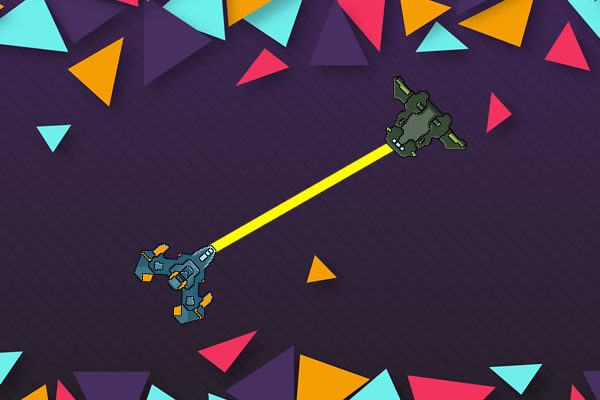# Get the angle between 2 objects in Phaser

Something that has been very useful for me lately is to be able to get the angle between two objects or get the angle between the mouse and a central character. For example in a game with a spaceship where you want to click and fire, you need to know the angle to be able to turn the ship. The actual math to get the angle is a bit complex, and I won’t pretend I understand it, but it has been a very useful snippet for me to have.

```getAngle: function(obj1, obj2) {
var angleRadians = Math.atan2(obj2.y - obj1.y, obj2.x - obj1.x);
// angle in degrees
var angleDeg = (Math.atan2(obj2.y - obj1.y, obj2.x - obj1.x) * 180 / Math.PI);
return angleDeg;
},```

All you need to do to get the angle is to pass two objects like this
var angle=this.getAngle(goodGuy,monster);

Here is an example where I use the angle between the mouse and a space ship to turn the ship.
Click anywhere to have the ship point at the mouse.

And here is the code for the example

```var StateMain = {
},
create: function() {
this.ship.anchor.set(0.5, 0.5);
},
clickCanvas: function() {
//make an object from the mouse postion
var obj1 = {
x: game.input.x,
y: game.input.y
};
//get the angle between the mouse and the ship and assign that to the
//ship's angle
this.ship.angle = this.getAngle(obj1, this.ship);
},
getAngle: function(obj1, obj2) {
//I use the offset because the ship is pointing down
//at the 6 o'clock position
//set to 0 if your sprite is facing 3 o'clock
//set to 180 if your sprite is facing 9 o'clock
//set to 270 if your sprite is facing 12 o'clock
//
offSet = 90;